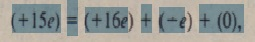# Beta Decay

Beta Decay

A nucleus that decays spontaneously by emitting an electron or a positron (a positively charged particle with the mass of an electron) is said to undergo beta decay. Like alpha decay, this is a spontaneous process, with a def nite  disintegration energy  and half-life. Again like alpha decay, beta decay is a statistical process, governedby Eqs. 43-14 d 43-15. In beta-minus ({3-) decay, an electron is emitted by a nucleus, as in the decayIn beta-plus ({3+) decay, a positron is emitted by a nucleus, as in the decayThe symbol I’ represents a neutrino, a neutral particle, with very. little or no mass, that is emitted from the nucleus along with the electron or positron during the decay  process. Neutrinos interact only very weakly with matter and-for that reasonable  so extremely difficult to detect that their presence long went unnoticed. Both charge and nucleon number are conserved in the above two processes. In the decay of Eq. 43-23, for example, we can write for charge conservation.because J2p has 15 protons. J2S has 16 protons, and the neutrino v has zero charge. Similarly, for nucleon conservation, we can write.because 32p and 32Seach have 32 nucleons and neither the electron nor the neutrino is a nucleon. It may seem surprising that nuclei can emit electrons, positrons, and neutrinos, since we have said that nuclei ar  made up of neutrons and protons only. However, we saw earlier that atoms emit photons, and we certainly do not say that atoms
“contain” photons. We say that the pho  ons are created during the emission process.It is the same with the electrons. positrons. and neutrinos emitted from nuclei during beta decay. They are created during the emission process. For beta-minus decay. a neutron transforms into a proton within the nucleus according to

n •…• p + e ” + v.

For beta-plus decay. a proton transforms into a neutron via

p ….•n + e ” + v.

Both of these beta-decay processes provide evidence that-as was pointed neutrons and protons are not truly fundamental particles. These processes show why the mass number A of a nuclide undergoing beta decay does not change; one of its constituent nucleons simply changes its character according to Eq. 43-25 or 43-26

In both alpha decay and beta decay, the same amount of energy is released in every individual decay of a particular radionuclide, In the alpha decay of a particular radionuclide, every emitted alpha particle has the same sharply defined kinetic energy,  However, in the beta-minus decay of Eq. 43-25 with electron emission t!:.:disintegration energy Q is shared-in varying proportions-between the emitter., electron and neutrino. Sometimes the electron gets nearly all the energy, sometimes the neutrino does. In every case, however, the sum of the electron’s energy and the
neutrino’s energy gives the same value Q. A similar sharing of energy, with a sum equal 10 Q, occurs in beta-plus decay (Eq. 43-26).  Thus, in beta decay the energy of the emitted electrons or positrons may range from zero up to a certain maximum Kmax• Figure 43-10 shows the distribution of positron energies for the beta decay of 64CU(see Eq. 43-24). The maximum positron energy KmaJ( must equal the disintegration energy Q because the neutrino carries
away:y approximately zero energ  when the positron carries away Kmax; that is,